Next:Important ObservationsUp:Characterizations of Generalized Entropies

Entropy of Order r and Degree s

Sharma and Mittal (1975)  presented an axiomatic characterization of entropy of order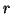and degree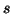. It is based on the Rényi's approach, where the additivity property has been changed (generally referred as nonadditivity).

Let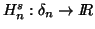be a real valued continuous function satisfying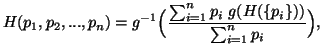where g is a strictly monotonic continuous function, and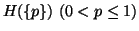is the self-information of an event of a probability distribution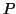satisfying:

(i)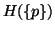is a continuous function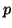in (0,1].
(ii)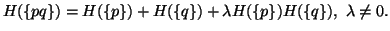(iii)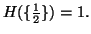Then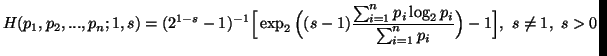(3.20)

and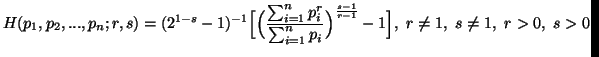(3.21)

Van der Pyl (1977) restructured the above axiomatic system and considered as follows:

Let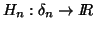be a real valued continuous function satisfying the following:

(i)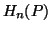is a symmetric function of its arguments.
(ii)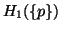is continuous in (0,1].
(iii)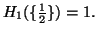(iv) There is a sequence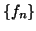such that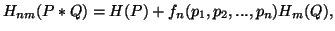for all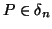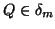and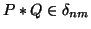.
(v) There exists a strictly monotonic continuous function g such that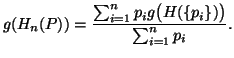Then the above set of axioms lead to the measures (3.20) and (3.21).

Picard (1979)  extended the above set of axioms by introducing the idea of weights or preferences and came up with the weighted entropies given in section 3.6.2.

The measure (3.21) can be characterized in a much more simplified form given as follows:

Let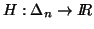be a real valued continuous function satisfying the following axioms:

(i)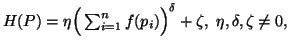where f is a continuous function defined on [0,1].
(ii)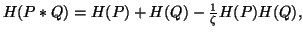for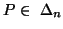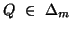and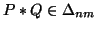.
(iii)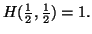Then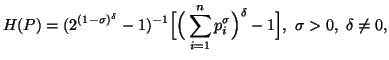that is same as (3.21) for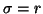and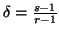, with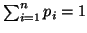.
21-06-2001
Inder Jeet Taneja
Departamento de Matemática - UFSC
88.040-900 Florianópolis, SC - Brazil